# Chapter 14:Dams and Retaining Walls¶

## Problem no 14.1,Page No.325¶

In :
import math
%matplotlib  inline

#Initilization of variables
b=2 #m #width
FOS=1.5 #Factor of safety
#rho_mason=2.5*rho_w
mu=0.5 #coeffeicient of friction

#Calculations

#Let L=1 m (length of dam)
L=1
#W=b*H*L*rho
#After substituting values and Further simplifying we get
#W=2*H*rho

#Total Pressure
#P=W*H**2*2**-1

x_bar=b*2**-1 #Distance of Line of action of W from waterface

#Distance of pt where resultant cuts the base measured from Line of action
#x=P*W**-1*H*3**-1
#After substituting values and Further simplifying we get
#x=H**2*30**-1

#x_bar+x=2*b*3**-1
#After substituting values and Further simplifying we get
#1+H**2*30**-1=2*b*3**-1
H=(30*(2*b*3**-1-1))**0.5 #height of dam

#Frictional Resistance offered at the base
#F=mu*W
#After substituting values and Further simplifying we get
#F=3.16*rho

#Total Lateral Pressure
#P=W*H**2*2**-1
#P=4.99*W

#Factor of safety against sliding
#FOS1=F*P**-1=3.16*4.99**-1*rho_mason*rho_w**-1
FOS1=3.16*4.99**-1*2.5

#FOS1>FOS

#Result
print"Dam is safe against sliding",round(FOS1,2)

Dam is safe against sliding 1.58


## Problem no 14.2,Page No.327¶

In :
import math
%matplotlib  inline

#Initilization of variables
D=2 #m #External Diameter
d=1.5 #m #Internal Diameter
P=1600 #N/m**2 #N/m**2 #Wind Pressure
W=19200 #N/m**2 #Weight of masonry

#Calculations

#Let H be max height of dam

#W2=pi*4**-1*(D**2-d**2)*H*W #weight of chimney
#W2=26400*H

#Eccentricrty
x=(D**2+d**2)*(8*D)**-1

#P2=H*D*P #Lateral thrust of wind on chimney
#P2=3200*H

#Now by using the relation we get P*W**-1=x*(H*2**-1)**-1
#After substituting values and Further simplifying we get
H=0.39*2*26400*3200**-1

#result
print"The Height of Dam is",round(H,2),"m"

The Height of Dam is 6.44 m


## Problem no 14.3,Page No.327¶

In :
import math
%matplotlib  inline

#Initilization of variables
rho_w=10 #KN/m**3 #Density of water
rho_mason=22.4 #KN/m**3 #Density of mason
H=6 #m #height of dam
a=1 #m #width of top
b=4 #m #bottom width
h=5.5 #m #Weight of water depth

#Calculations

#Let L=1 m (length of dam)
L=1

#weight of dam
W=(a+b)*2**-1*H*a*rho_mason

#Lateral thrust
P=rho_w*h**2*a*2**-1

#distance of Line of action of W measured from vertical face
x_bar=(b**2+b*a+a**2)*(3*(b+a))**-1

#distance of pt where resultant cuts the base
x=P*W**-1*h*3**-1

#Eccentricity
e=x_bar+x-b*2**-1

#Stress at Pt B
sigma1=W*b**-1*(1-6*e*b**-1)

#stress at Pt C
sigma2=W*b**-1*(1+6*e*b**-1)

#Result
print"Max stress intensities at the base is",round(sigma2,2),"KN/m**2"
print"Min stress intensities at the base is",round(sigma1,2),"KN/m**2"

#Plotting the Shear Force Diagram

X1=[0,L,L]
Y1=[sigma2,sigma1,0]
Z1=[0,0,0]
plt.plot(X1,Y1,X1,Z1)
plt.xlabel("Length x in m")
plt.ylabel("Shear Force in kN")
plt.show()

Max stress intensities at the base is 112.38 KN/m**2
Min stress intensities at the base is 55.62 KN/m**2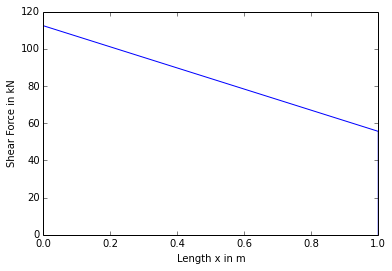## Problem no 14.4,Page No.329¶

In :
import math
%matplotlib  inline

#Initilization of variables
H=10 #m #height od dam
a=2 #m #top width
b=5 #m #bottom width
W=25 #KN/m**3 #weight of mason
rho_w=10 #KN/m**3 #density of water

#Calculations

#Let L=1 m (length of dam)
L=1

#weight of dam
W2=(b+a)*H*L*W*2**-1

##Lateral thrust
P=rho_w*H**2*L*2**-1

#Resultant thrust
R=(P**2+W**2)**0.5

#Distance of Line of action from vertical base
x_bar=(b**2+b*a+a**2)*(3*(b+a))**-1

##distance of pt where resultant cuts the base
x=P*W2**-1*H*3**-1

#Eccentricity
e=x_bar+x-b*2**-1

#Stress at Pt B
sigma1=W2*b**-1*(1-6*e*b**-1)

#stress at Pt C
sigma2=W2*b**-1*(1+6*e*b**-1)

#Result
print"The Resultant Thrust on the base is",round(R,2),"KN"

#Plotting the Shear Force Diagram

X1=[0,L,L]
Y1=[-sigma2,-sigma1,0]
Z1=[0,0,0]
plt.plot(X1,Y1,X1,Z1)
plt.xlabel("Length x in m")
plt.ylabel("Shear Force in kN")
plt.show()

The Resultant Thrust on the base is 500.62 KN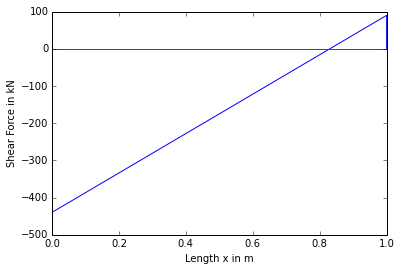## Problem no 14.5,Page No.330¶

In :
import math
%matplotlib  inline

#Initilization of variables
H=30 #m #height of Dam
h=29 #m #height of water
rho_w=9810 #N/m**3
rho_mason=22560 #N/m**3
sigma1=0 #KN/m**3
sigma2=880 #KN/m**3

#Calculations

#Let L=1 m (length of dam)
L=1

#weight of dam
#W=(a+b)*2**-1*L*H*rho_mason*10**-3
#After substituting values and Further simplifying we get
#W=338.4*(a+b) #equation1

#Pressure at B=0, Sinc etension at base has just been avoided

#Eccentricity
e=b*6**-1  #as sigma1=0

#Pressure at C
#sigma2=W2*b**-1*(1+6*e*b**-1)
#After substituting values and Further simplifying we get
#W=440*b

#From equation1,440*b=338*(a+b)
#b=3.33*a

#the distance of C.Gof dam
#x_bar=(b**2+b*a+a**2)*(3*(b+a))**-1
#After substituting values and Further simplifying we get
#x_bar=1.187*a

#distance of pt where resultant cuts the base
#x=P*W2**-1*H*3**-1
#After substituting values and Further simplifying we get
#x=27.214*a**-1

#Now x_bar+x=2*3**-1*b
#After substituting values and Further simplifying we get
a=(27.17*(2.22-1.187)**-1)**0.5
b=3.33*a

#Result
print"The top width dam is",round(a,2),"m"
print"The bottom width dam is",round(b,2),"m"

#Plotting the Shear Force Diagram

X1=[0,L,L]
Y1=[sigma2,sigma1,0]
Z1=[0,0,0]
plt.plot(X1,Y1,X1,Z1)
plt.xlabel("Length x in m")
plt.ylabel("Shear Force in kN")
plt.show()

The top width dam is 5.13 m
The bottom width dam is 17.08 m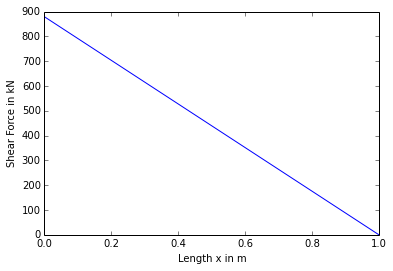## Problem no 14.6,Page No.332¶

In :
import math
%matplotlib  inline

#Initilization of variables
H= 4 #m #height of dam
a=1 #m #Top width
b=3 #m #bottom width
rho1=9810 #N/m**3 #weight of water
rho2=19620 #n/m**3 #Weight of mason

#Calculations

#Let L=1 m (length of dam)
L=1

#weight of dam
W=(a+b)*2**-1*L*H*rho2*10**-3

##Lateral thrust
P=rho1*H**2*L*2**-1*10**-3

#Distance of Line of action from vertical base
x_bar=(b**2+b*a+a**2)*(3*(b+a))**-1

#distance of pt where resultant cuts the base
x=P*W**-1*H*3**-1

#Eccentricity
e=x_bar+x-b*2**-1

#Stress at Pt B
sigma1=W*10**3*b**-1*(1-6*e*b**-1)

#stress at Pt C
sigma2=W*10**3*b**-1*(1+6*e*b**-1)

#Stresses at the base when resorvoir is empty

e2=x_bar-b*2**-1

#Stress at C
sigma2_2=W*10**3*b**-1*(1+6*round(e2,2)*b**-1)

#Stress at Pt B
sigma1_2=W*10**3*b**-1*(1-6*round(e2,2)*b**-1)

#result
print"When the Reservoir is full :sigma1",round(sigma1,2),"KN/m**2"
print"                           :sigma2",round(sigma2,2),"KN/m**2"
print"When the Reservoir is empty:sigma1_2",round(sigma1_2,2),"KN/m**2"
print"                           :sigma2_2",round(sigma2_2,2),"KN/m**2"

When the Reservoir is full :sigma1 26160.0 KN/m**2
:sigma2 78480.0 KN/m**2
When the Reservoir is empty:sigma1_2 96268.8 KN/m**2
:sigma2_2 8371.2 KN/m**2


## Problem no 14.7,Page No.333¶

In :
import math
%matplotlib  inline

#Initilization of variables
H=8 #m #height of dam
h=7.5 #m #Height of water
a=1 #m #top width
mu=0.6 #Coeffeicient of friction
rho_mason=22.4 #KN/m**3 #weight of mason
rho_w=9.81 #KN/m**3 #density of water

#Calculations

#weight of dam
#W=(a+b)*2**-1*L*H*rho2*10**-3
#After substituting values and further simplifying we get
#W=89600*(b+1)

#Distance of Line of action from vertical base
#x_bar=(b**2+b*a+a**2)*(3*(b+a))**-1
#After substituting values and further simplifying we get
#x_bar=(1+b+b**2)*(3*(1+b))**-1

#Lateral thrust
P=rho_w*h**2*2**-1

#Min width to avoid tension at base
#Z=x_bar+P*W**-1*h*3**-1
#Z=2*3**-1*b
#After substituting values and further simplifying we get
#b**2+b-24.09=0
a=1
b=1
c=-24.09

X=b**2-4*a*c

b1=(-b+X**0.5)*(2*a)**-1
b2=(-b-X**0.5)*(2*a)**-1

#Thus width cannot be negative,b1 is considered

#Min width to avoid sliding
#mu*W>P
#After substituting values and further simplifying we get
b=P*10**3*(mu*89600)**-1-1

#Result
print"The Min bottom width is",round(b,2),"m"

The Min bottom width is 4.13 m


## Problem no 14.8,Page No.334¶

In :
import math
%matplotlib  inline

#Initilization of variables
H=10 #m #height of dam
a=1 #m #top width
b=7 #m #Bottom width
rho_mason=19620 #N/m**3 #weight of mason
rho_w=9810 #N/m**3 #density of water

#Calculations

#Lateral thrust
P=rho_w*H**2*2**-1

#weight of dam
W=(rho_w*H*2**-1*a)+(rho_mason*(a+b)*2**-1*H)

#Taking Moment at B,M_B=0
x_bar=((rho_w*H*2**-1*1*3**-1)+(rho_mason*H*2**-1*2*3**-1)+(rho_mason*H*1.5)+(rho_mason*H*5*11*2**-1*3**-1))*W**-1

#Now using relation we get
x=P*W**-1*H*3**-1

#Eccentricity
e=x_bar+x-b*2**-1

#Max stress
sigma_max=W*b**-1*(1+6*round(e,2)*b**-1)

#Min stress
sigma_min=W*b**-1*(1-6*round(e,2)*b**-1)

#Result
print"The Max stresses on the base is",round(sigma_max,2),"N/m**2"
print"The Min stresses on the base is",round(sigma_min,2),"N/m**2"

The Max stresses on the base is 228372.8 N/m**2
The Min stresses on the base is 9870.06 N/m**2


## Problem no 14.10,Page No.337¶

In :
import math
%matplotlib  inline

#Initilization of variables
H=6 #m #height of dam
a=1 #m #top width
b=3 #m #Bottom width
rho_mason=22 #KN/m**3 #weight of mason
rho_earth=16 #KN/m**3 #density of water
phi=30 #Degree #angle of repose
mu=0.5 #Coeffecient of friction

#Calculations

#Let Length of dam ,L=1 m
L=1 #m

#weight of dam
W=(a+b)*2**-1*L*H*rho_mason

#Lateral thrust
P=rho_earth*H**2*L*2**-1*((1-sin(30*pi*180**-1))*(1+sin(phi*pi*180**-1))**-1)

#Distance of Line of action from vertical base
x_bar=(b**2+b*a+a**2)*(3*(b+a))**-1

#distance of pt where resultant cuts the base
x=P*W**-1*H*3**-1

#Eccentricity
e=x_bar+x-b*2**-1
e_max=b*6**-1

#stress at toe
sigma2=W*10**3*b**-1*(1+6*round(e,2)*b**-1)*10**-3

#Safe agaainst bearing

#Frictional Resistance
F=mu*W

#Hence F>P,so it is safe against sliding

#Result
print"Safe against bearing as well as sliding"

Safe against bearing as well as sliding


## Problem no 14.11,Page No.338¶

In :
import math
%matplotlib  inline

#Initilization of variables
H=8 #m #height of dam
a=1 #m #top width
b=4.5 #m #Bottom width
rho_mason=24 #KN/m**3 #weight of mason
rho_earth=20 #KN/m**3 #density of water
phi=30 #Degree #angle of repose
mu=0.5 #Coeffecient of friction
BC=120 #KN/m**2

#Calculations

#Let Length of dam ,L=1 m
L=1 #m

#weight of dam
W=(a+b)*2**-1*L*H*rho_mason

#Rankine's coeff earth pressure
K=((1-sin(30*pi*180**-1))*(1+sin(phi*pi*180**-1))**-1)

#Lateral thrust
P=rho_earth*H**2*L*2**-1*round(K,2)

#Distance of Line of action from vertical base
x_bar=(b**2+b*a+a**2)*(3*(b+a))**-1

#distance of pt where resultant cuts the base
x=P*W**-1*H*3**-1

#Eccentricity
e=x_bar+x-b*2**-1

#Pressure at heel B
sigma1=W*b**-1*(1-6*round(e,2)*b**-1)

#Pressure at heel C
sigma2=W*b**-1*(1+6*round(e,2)*b**-1)

#sigma2>120 #KN/m**2,so it is unsafe against bearing capacity of the soil

#result
print"Unsafe against the bearing capacity of soil"

#Plotting the Shear Force Diagram

X1=[0,L,L]
Y1=[sigma2,sigma1,0]
Z1=[0,0,0]
plt.plot(X1,Y1,X1,Z1)
plt.xlabel("Length x in m")
plt.ylabel("Shear Force in kN")
plt.show()

Unsafe against the bearing capacity of soil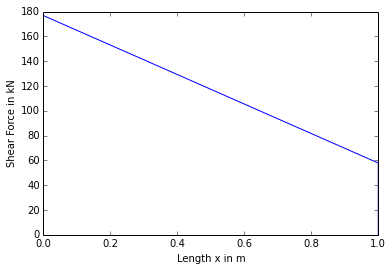## Problem no 14.12,Page No.340¶

In :
import math
%matplotlib  inline

#Initilization of variables
H=6 #m #height of dam
a=1.5 #m #top width
b=3.5 #m #Bottom width
rho_s=16 #KN/m**3 #density of soil
rho_mason=22.5 #KN/m**3 #density of mason
phi=30 #Degree #angle of repose

#Calculations

#Let Length of dam ,L=1 m
L=1 #m

#weight of dam
W=(a+b)*2**-1*L*H*rho_mason

#Rankine's coeff earth pressure
K=((1-sin(30*pi*180**-1))*(1+sin(phi*pi*180**-1))**-1)

#Lateral thrust
P=rho_s*H**2*L*2**-1*K

#Distance of Line of action from vertical base
x_bar=(b**2+b*a+a**2)*(3*(b+a))**-1

#distance of pt where resultant cuts the base
x=P*W**-1*H*3**-1

#Eccentricity
e=x_bar+x-b*2**-1

#Pressure at heel B
sigma1=W*b**-1*(1-6*round(e,3)*b**-1)

#Pressure at heel C
sigma2=W*b**-1*(1+6*round(e,3)*b**-1)

#Result
print"The Max Intensities of soil at the wall is",round(sigma2,2),"KN/m**2"
print"The Min Intensities of soil at the wall is",round(sigma1,2),"KN/m**2"

#Plotting the Shear Force Diagram

X1=[0,L,L]
Y1=[sigma2,sigma1,0]
Z1=[0,0,0]
plt.plot(X1,Y1,X1,Z1)
plt.xlabel("Length x in m")
plt.ylabel("Shear Force in kN")
plt.show()

The Max Intensities of soil at the wall is 118.91 KN/m**2
The Min Intensities of soil at the wall is 73.95 KN/m**2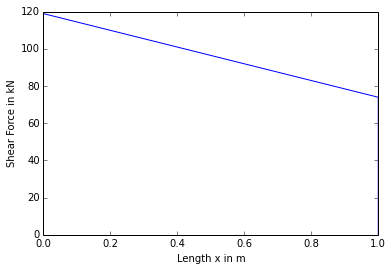## Problem no 14.13,Page No.341¶

In :
import math
%matplotlib  inline

#Initilization of variables
H=6 #m #height of dam
a=1 #m #top width
b=3 #m #Bottom width
rho_s=18 #KN/m**3 #density of soil
rho_mason=24 #KN/m**3 #density of mason
alpha=20
phi=30

#Calculations

#Let Length of dam ,L=1 m
L=1 #m

a2=cos(alpha*pi*180**-1)
b2=(cos(alpha*pi*180**-1)-((cos(alpha*pi*180**-1)**2-cos(phi*pi*180**-1)**2))**0.5)
c2=(cos(alpha*pi*180**-1)+((cos(alpha*pi*180**-1)**2-cos(phi*pi*180**-1)**2)**0.5))

X=a2*b2*c2**-1

#Total Pressue on the wall
P=rho_s*H**2*2**-1*X

#The Horizontal component of pressure
P_H=P*cos(20*pi*180**-1)

#The Vertical component of pressure
P_V=P*sin(20*pi*180**-1)

#weight of wall
W=(a+b)*H*rho_mason*2**-1

#TotaL Weight
W1=W+P_V

x_bar=(rho_mason*a*H*0.5+rho_mason*H*2)*W1**-1

x=P_H*W1**-1*H*3**-1

#eccentricity
e=x_bar+x-b*2**-1

#Stress at the toe at C
sigma_max=W1*b**-1*(1+6*round(e,2)*b**-1)

#Stress at the heel at B
sigma_min=W1*b**-1*(1-6*round(e,2)*b**-1)

#Result
print"Pressure at the base of the wall:Pressure at the heel",round(sigma_min,2),"KN/m**2"
print"                                :Pressure at the toe",round(sigma_max,2),"KN/m**2"

Pressure at the base of the wall:Pressure at the heel 37.84 KN/m**2
:Pressure at the toe 184.76 KN/m**2